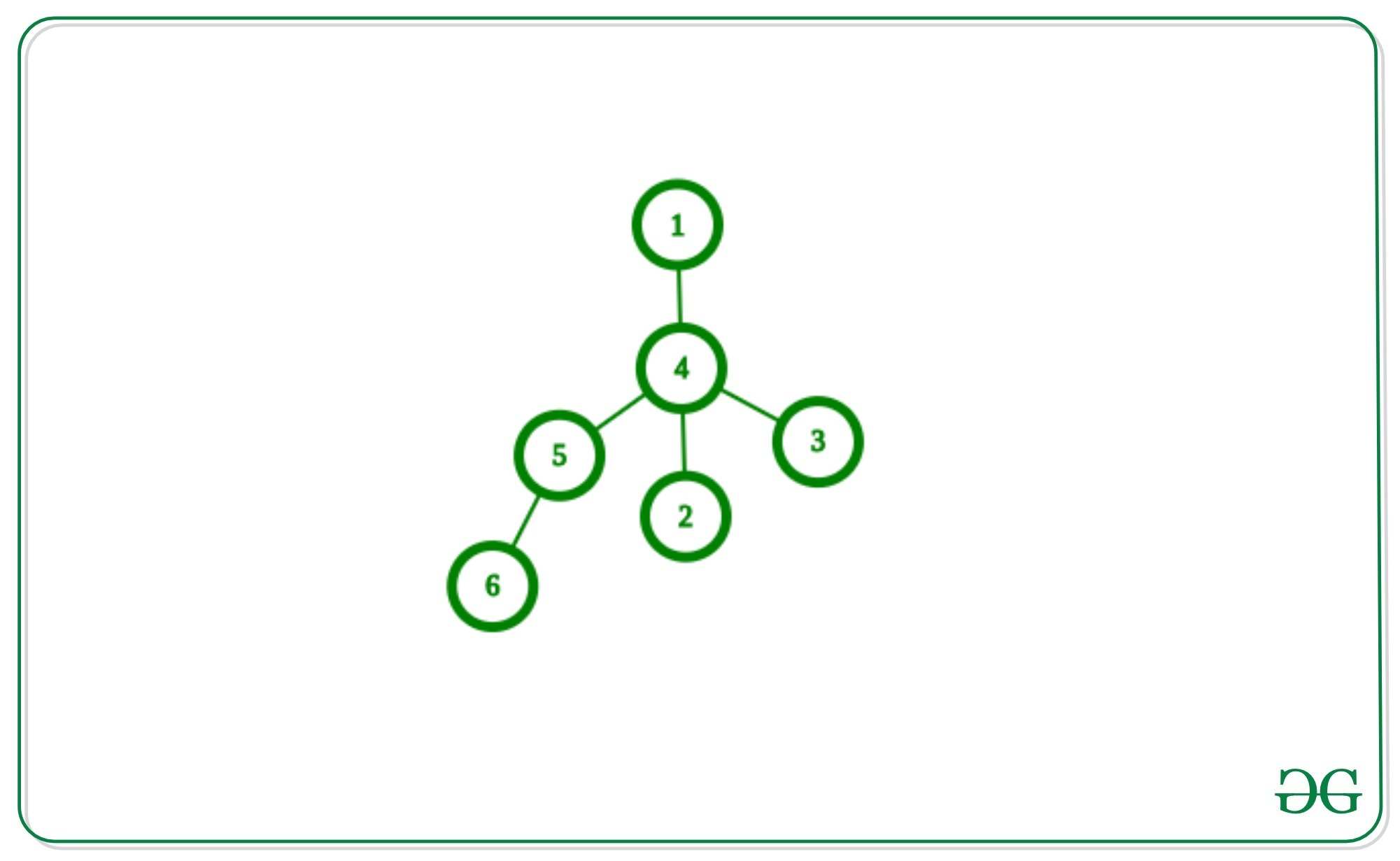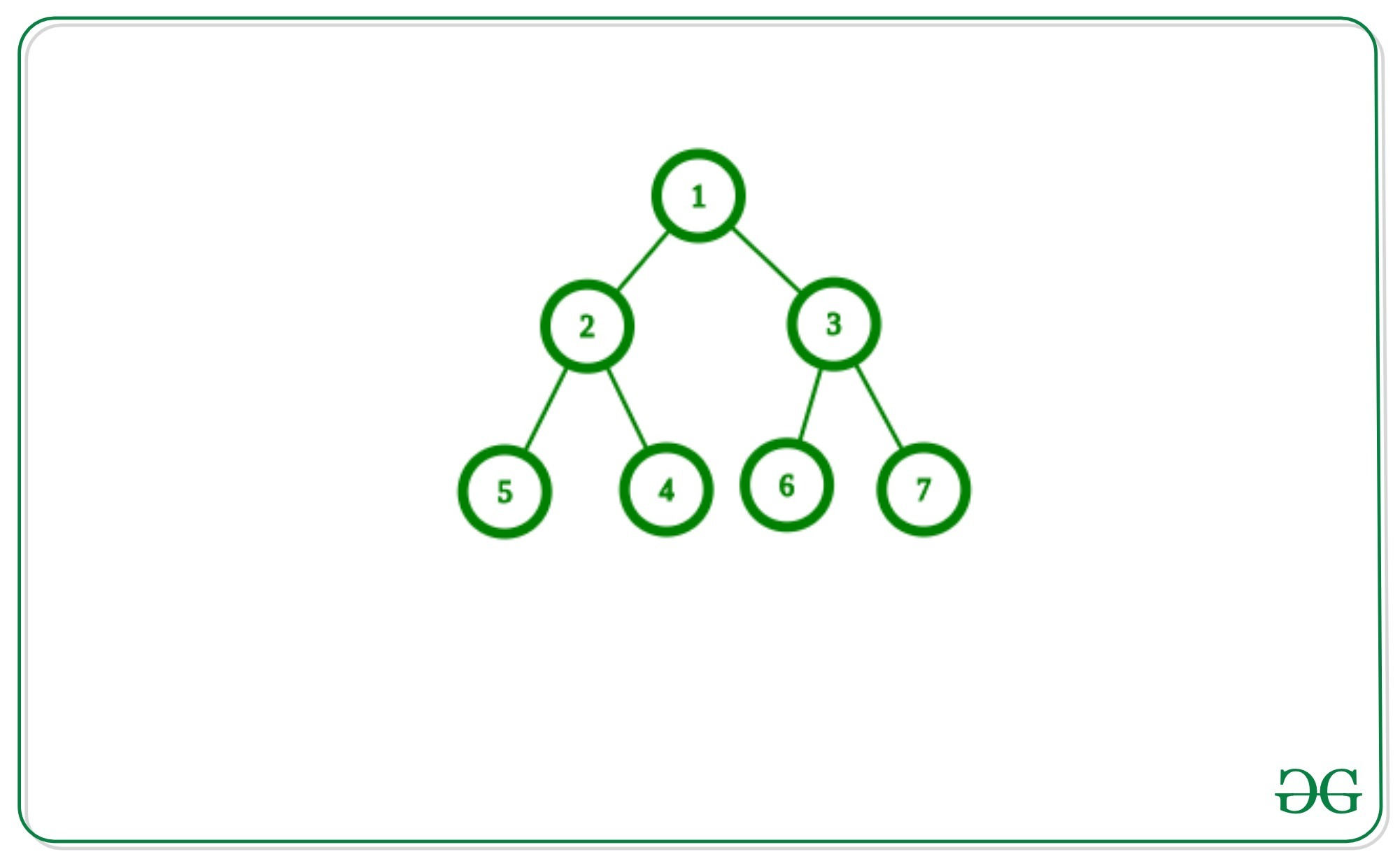Related Articles
Find farthest node from each node in Tree
• Difficulty Level : Medium
• Last Updated : 29 Dec, 2020

Given a Tree, the task is to find the farthest node from each node to another node in this tree.

Example:

Input: Given Adjacency List of Below Tree:Output:
Farthest node from node 1: 6
Farthest node from node 2: 6
Farthest node from node 3: 6
Farthest node from node 4: 6
Farthest node from node 5: 1
Farthest node from node 6: 1

Input:Output:
Farthest node from node 1: 4
Farthest node from node 2: 7
Farthest node from node 3: 4
Farthest node from node 4: 7
Farthest node from node 5: 7
Farthest node from node 6: 4
Farthest node from node 7: 4

Approach:
First, we have to find two end vertices of the diameter and to find that, we will choose an arbitrary vertex and find the farthest node from this arbitrary vertex and this node will be one end of the diameter and then make it root to find farthest node from it, which will be the other end of diameter. Now for each node, the farthest node will be one of these two end vertices of the diameter of the tree.

Why it works?
Let x and y are the two end vertices of the diameter of the tree and a random vertex is u. Let the farthest vertex from u is v, not x or y. As v is the farthest from u then a new diameter will form having end vertices as x, v or y, v which has greater length but a tree has a unique length of the diameter, so it is not possible and the farthest vertex from u must be x or y.

Below is the implementation of above approach:

## C++

 `// C++ implementation to find the``// farthest node from each vertex``// of the tree` `#include ` `using` `namespace` `std;` `#define N 10000` `// Adjacency list to store edges``vector<``int``> adj[N];` `int` `lvl[N], dist1[N], dist2[N];` `// Add edge between``// U and V in tree``void` `AddEdge(``int` `u, ``int` `v)``{``    ``// Edge from U to V``    ``adj[u].push_back(v);``    ` `    ``// Edge from V to U``    ``adj[v].push_back(u);``}` `int` `end1, end2, maxi;` `// DFS to find the first``// End Node of diameter``void` `findFirstEnd(``int` `u, ``int` `p)``{``    ``// Calculating level of nodes``    ``lvl[u] = 1 + lvl[p];``    ``if` `(lvl[u] > maxi) {``        ``maxi = lvl[u];``        ``end1 = u;``    ``}``    ` `    ``for` `(``int` `i = 0; i < adj[u].size(); i++) {``        ` `        ``// Go in opposite``        ``// direction of parent``        ``if` `(adj[u][i] != p) {``            ``findFirstEnd(adj[u][i], u);``        ``}``    ``}``}` `// Function to clear the levels``// of the nodes``void` `clear(``int` `n)``{``    ``// set all value of lvl[]``    ``// to 0 for next dfs``    ``for` `(``int` `i = 0; i <= n; i++) {``        ``lvl[i] = 0;``    ``}``    ` `    ``// Set maximum with 0``    ``maxi = 0;``    ``dist1 = dist2 = -1;``}` `// DFS will calculate second``// end of the diameter``void` `findSecondEnd(``int` `u, ``int` `p)``{``    ``// Calculating level of nodes``    ``lvl[u] = 1 + lvl[p];``    ``if` `(lvl[u] > maxi) {``        ``maxi = lvl[u];``        ` `        ``// Store the node with``        ``// maximum depth from end1``        ``end2 = u;``    ``}` `    ``for` `(``int` `i = 0; i < adj[u].size(); i++) {``        ``// Go in opposite``        ``// direction of parent``        ``if` `(adj[u][i] != p) {``            ``findSecondEnd(adj[u][i], u);``        ``}``    ``}``}` `// Function to find the distance``// of the farthest distant node``void` `findDistancefromFirst(``int` `u, ``int` `p)``{``    ``// Storing distance from``    ``// end1 to node u``    ``dist1[u] = 1 + dist1[p];``    ``for` `(``int` `i = 0; i < adj[u].size(); i++) {``        ``if` `(adj[u][i] != p) {``            ``findDistancefromFirst(adj[u][i], u);``        ``}``    ``}``}` `// Function to find the distance``// of nodes from second end of diameter``void` `findDistancefromSecond(``int` `u, ``int` `p)``{``    ``// storing distance from end2 to node u``    ``dist2[u] = 1 + dist2[p];``    ``for` `(``int` `i = 0; i < adj[u].size(); i++) {``        ``if` `(adj[u][i] != p) {``            ``findDistancefromSecond(adj[u][i], u);``        ``}``    ``}``}` `void` `findNodes(){``    ``int` `n = 5;` `    ``// Joining Edge between two``    ``// nodes of the tree``    ``AddEdge(1, 2);``    ``AddEdge(1, 3);``    ``AddEdge(3, 4);``    ``AddEdge(3, 5);` `    ``// Find the one end of``    ``// the diameter of tree``    ``findFirstEnd(1, 0);``    ``clear(n);``    ` `    ``// Find the other end``    ``// of the diameter of tree``    ``findSecondEnd(end1, 0);` `    ``// Find the distance``    ``// to each node from end1``    ``findDistancefromFirst(end1, 0);` `    ``// Find the distance to``    ``// each node from end2``    ``findDistancefromSecond(end2, 0);` `    ``for` `(``int` `i = 1; i <= n; i++) {``        ``int` `x = dist1[i];``        ``int` `y = dist2[i];``        ` `        ``// Comparing distance between``        ``// the two ends of diameter``        ``if` `(x >= y) {``            ``cout << end1 << ``' '``;``        ``}``        ``else` `{``            ``cout << end2 << ``' '``;``        ``}``    ``}``}` `// Driver code``int` `main()``{``    ``// Function Call``    ``findNodes();` `    ``return` `0;``}`

## Java

 `// Java implementation to find the``// farthest node from each vertex``// of the tree``import` `java.util.*;` `class` `GFG{` `static` `int` `N = ``10000``;` `// Adjacency list to store edges``@SuppressWarnings``(``"unchecked"``)``static` `Vector[] adj = ``new` `Vector[N];` `static` `int``[] lvl = ``new` `int``[N],``           ``dist1 = ``new` `int``[N],``           ``dist2 = ``new` `int``[N];` `// Add edge between``// U and V in tree``static` `void` `AddEdge(``int` `u, ``int` `v)``{``    ` `    ``// Edge from U to V``    ``adj[u].add(v);` `    ``// Edge from V to U``    ``adj[v].add(u);``}` `static` `int` `end1, end2, maxi;` `// DFS to find the first``// End Node of diameter``static` `void` `findFirstEnd(``int` `u, ``int` `p)``{``    ` `    ``// Calculating level of nodes``    ``lvl[u] = ``1` `+ lvl[p];``    ``if` `(lvl[u] > maxi)``    ``{``        ``maxi = lvl[u];``        ``end1 = u;``    ``}` `    ``for``(``int` `i = ``0``; i < adj[u].size(); i++)``    ``{``        ` `        ``// Go in opposite``        ``// direction of parent``        ``if` `(adj[u].elementAt(i) != p)``        ``{``            ``findFirstEnd(adj[u].elementAt(i), u);``        ``}``    ``}``}` `// Function to clear the levels``// of the nodes``static` `void` `clear(``int` `n)``{``    ` `    ``// Set all value of lvl[]``    ``// to 0 for next dfs``    ``for``(``int` `i = ``0``; i <= n; i++)``    ``{``        ``lvl[i] = ``0``;``    ``}` `    ``// Set maximum with 0``    ``maxi = ``0``;``    ``dist1[``0``] = dist2[``0``] = -``1``;``}` `// DFS will calculate second``// end of the diameter``static` `void` `findSecondEnd(``int` `u, ``int` `p)``{``    ` `    ``// Calculating level of nodes``    ``lvl[u] = ``1` `+ lvl[p];``    ``if` `(lvl[u] > maxi)``    ``{``        ``maxi = lvl[u];` `        ``// Store the node with``        ``// maximum depth from end1``        ``end2 = u;``    ``}` `    ``for``(``int` `i = ``0``; i < adj[u].size(); i++)``    ``{``        ` `        ``// Go in opposite``        ``// direction of parent``        ``if` `(adj[u].elementAt(i) != p)``        ``{``            ``findSecondEnd(adj[u].elementAt(i), u);``        ``}``    ``}``}` `// Function to find the distance``// of the farthest distant node``static` `void` `findDistancefromFirst(``int` `u, ``int` `p)``{``    ` `    ``// Storing distance from``    ``// end1 to node u``    ``dist1[u] = ``1` `+ dist1[p];``    ``for``(``int` `i = ``0``; i < adj[u].size(); i++)``    ``{``        ``if` `(adj[u].elementAt(i) != p)``        ``{``            ``findDistancefromFirst(``                ``adj[u].elementAt(i), u);``        ``}``    ``}``}` `// Function to find the distance``// of nodes from second end of diameter``static` `void` `findDistancefromSecond(``int` `u, ``int` `p)``{``    ` `    ``// Storing distance from end2 to node u``    ``dist2[u] = ``1` `+ dist2[p];``    ``for``(``int` `i = ``0``; i < adj[u].size(); i++)``    ``{``        ``if` `(adj[u].elementAt(i) != p)``        ``{``            ``findDistancefromSecond(``                ``adj[u].elementAt(i), u);``        ``}``    ``}``}` `static` `void` `findNodes()``{``    ``int` `n = ``5``;` `    ``// Joining Edge between two``    ``// nodes of the tree``    ``AddEdge(``1``, ``2``);``    ``AddEdge(``1``, ``3``);``    ``AddEdge(``3``, ``4``);``    ``AddEdge(``3``, ``5``);` `    ``// Find the one end of``    ``// the diameter of tree``    ``findFirstEnd(``1``, ``0``);``    ``clear(n);` `    ``// Find the other end``    ``// of the diameter of tree``    ``findSecondEnd(end1, ``0``);` `    ``// Find the distance``    ``// to each node from end1``    ``findDistancefromFirst(end1, ``0``);` `    ``// Find the distance to``    ``// each node from end2``    ``findDistancefromSecond(end2, ``0``);` `    ``for``(``int` `i = ``1``; i <= n; i++)``    ``{``        ``int` `x = dist1[i];``        ``int` `y = dist2[i];` `        ``// Comparing distance between``        ``// the two ends of diameter``        ``if` `(x >= y)``        ``{``            ``System.out.print(end1 + ``" "``);``        ``}``        ``else``        ``{``            ``System.out.print(end2 + ``" "``);``        ``}``    ``}``}` `// Driver Code``public` `static` `void` `main(String[] args)``{``    ``for``(``int` `i = ``0``; i < N; i++)``    ``{``        ``adj[i] = ``new` `Vector<>();``    ``}` `    ``// Function call``    ``findNodes();``}``}` `// This code is contributed by sanjeev2552`

## Python3

 `# Python3 implementation to find the``# farthest node from each vertex``# of the tree`` ` `# Add edge between``# U and V in tree``def` `AddEdge(u, v):``    ` `    ``global` `adj``    ` `    ``# Edge from U to V``    ``adj[u].append(v)`` ` `    ``# Edge from V to U``    ``adj[v].append(u)`` ` `# DFS to find the first``# End Node of diameter``def` `findFirstEnd(u, p):``    ` `    ``global` `lvl, adj, end1, maxi``    ` `    ``# Calculating level of nodes``    ``lvl[u] ``=` `1` `+` `lvl[p]``    ` `    ``if` `(lvl[u] > maxi):``        ``maxi ``=` `lvl[u]``        ``end1 ``=` `u`` ` `    ``for` `i ``in` `range``(``len``(adj[u])):`` ` `        ``# Go in opposite``        ``# direction of parent``        ``if` `(adj[u][i] !``=` `p):``            ``findFirstEnd(adj[u][i], u)`` ` `# Function to clear the levels``# of the nodes``def` `clear(n):``    ` `    ``global` `lvl, dist1, dist2``    ` `    ``# Set all value of lvl[]``    ``# to 0 for next dfs``    ``for` `i ``in` `range``(n ``+` `1``):``        ``lvl[i] ``=` `0`` ` `    ``# Set maximum with 0``    ``maxi ``=` `0``    ``dist1[``0``] ``=` `dist2[``0``] ``=` `-``1`` ` `# DFS will calculate second``# end of the diameter``def` `findSecondEnd(u, p):``    ` `    ``global` `lvl, adj, maxi, end2``    ` `    ``# Calculating level of nodes``    ``lvl[u] ``=` `1` `+` `lvl[p]``    ` `    ``if` `(lvl[u] > maxi):``        ``maxi ``=` `lvl[u]`` ` `        ``# Store the node with``        ``# maximum depth from end1``        ``end2 ``=` `u`` ` `    ``for` `i ``in` `range``(``len``(adj[u])):``        ` `        ``# Go in opposite``        ``# direction of parent``        ``if` `(adj[u][i] !``=` `p):``            ``findSecondEnd(adj[u][i], u)`` ` `# Function to find the distance``# of the farthest distant node``def` `findDistancefromFirst(u, p):``    ` `    ``global` `dist1, adj``    ` `    ``# Storing distance from``    ``# end1 to node u``    ``dist1[u] ``=` `1` `+` `dist1[p]``    ` `    ``for` `i ``in` `range``(``len``(adj[u])):``        ``if` `(adj[u][i] !``=` `p):``            ``findDistancefromFirst(adj[u][i], u)`` ` `# Function to find the distance``# of nodes from second end of diameter``def` `findDistancefromSecond(u, p):``    ` `    ``global` `dist2, adj``    ` `    ``# Storing distance from end2 to node u``    ``dist2[u] ``=` `1` `+` `dist2[p]``    ` `    ``for` `i ``in` `range``(``len``(adj[u])):``        ``if` `(adj[u][i] !``=` `p):``            ``findDistancefromSecond(adj[u][i], u)`` ` `def` `findNodes():``    ` `    ``global` `adj, lvl, dist1, dist2, end1, end2, maxi``    ``n ``=` `5`` ` `    ``# Joining Edge between two``    ``# nodes of the tree``    ``AddEdge(``1``, ``2``)``    ``AddEdge(``1``, ``3``)``    ``AddEdge(``3``, ``4``)``    ``AddEdge(``3``, ``5``)`` ` `    ``# Find the one end of``    ``# the diameter of tree``    ``findFirstEnd(``1``, ``0``)``    ``clear(n)`` ` `    ``# Find the other end``    ``# of the diameter of tree``    ``findSecondEnd(end1, ``0``)`` ` `    ``# Find the distance``    ``# to each node from end1``    ``findDistancefromFirst(end1, ``0``)`` ` `    ``# Find the distance to``    ``# each node from end2``    ``findDistancefromSecond(end2, ``0``)`` ` `    ``for` `i ``in` `range``(``1``, n ``+` `1``):``        ``x ``=` `dist1[i]``        ``y ``=` `dist2[i]`` ` `        ``# Comparing distance between``        ``# the two ends of diameter``        ``if` `(x >``=` `y):``            ``print``(end1, end ``=` `" "``)``        ``else``:``            ``print``(end2, end ``=` `" "``)`` ` `# Driver code``if` `__name__ ``=``=` `'__main__'``:``    ` `    ``adj ``=` `[[] ``for` `i ``in` `range``(``10000``)]``    ``lvl ``=` `[``0` `for` `i ``in` `range``(``10000``)]``    ``dist1 ``=` `[``-``1` `for` `i ``in` `range``(``10000``)]``    ``dist2 ``=` `[``-``1` `for` `i ``in` `range``(``10000``)]``    ``end1, end2, maxi ``=` `0``, ``0``, ``0``    ` `    ``# Function Call``    ``findNodes()` `# This code is contributed by mohit kumar 29`

## C#

 `// C# implementation to find the``// farthest node from each vertex``// of the tree``using` `System;``using` `System.Collections.Generic;` `class` `GFG{` `static` `int` `N = 10000;` `// Adjacency list to store edges``static` `List<``int``>[] adj = ``new` `List<``int``>[N];` `static` `int``[] lvl = ``new` `int``[N],``           ``dist1 = ``new` `int``[N],``           ``dist2 = ``new` `int``[N];` `// Add edge between``// U and V in tree``static` `void` `AddEdge(``int` `u, ``int` `v)``{``    ` `    ``// Edge from U to V``    ``adj[u].Add(v);` `    ``// Edge from V to U``    ``adj[v].Add(u);``}` `static` `int` `end1, end2, maxi;` `// DFS to find the first``// End Node of diameter``static` `void` `findFirstEnd(``int` `u, ``int` `p)``{``    ` `    ``// Calculating level of nodes``    ``lvl[u] = 1 + lvl[p];``    ` `    ``if` `(lvl[u] > maxi)``    ``{``        ``maxi = lvl[u];``        ``end1 = u;``    ``}` `    ``for``(``int` `i = 0; i < adj[u].Count; i++)``    ``{``        ` `        ``// Go in opposite``        ``// direction of parent``        ``if` `(adj[u][i] != p)``        ``{``            ``findFirstEnd(adj[u][i], u);``        ``}``    ``}``}` `// Function to clear the levels``// of the nodes``static` `void` `clear(``int` `n)``{``    ` `    ``// Set all value of lvl[]``    ``// to 0 for next dfs``    ``for``(``int` `i = 0; i <= n; i++)``    ``{``        ``lvl[i] = 0;``    ``}` `    ``// Set maximum with 0``    ``maxi = 0;``    ``dist1 = dist2 = -1;``}` `// DFS will calculate second``// end of the diameter``static` `void` `findSecondEnd(``int` `u, ``int` `p)``{``    ` `    ``// Calculating level of nodes``    ``lvl[u] = 1 + lvl[p];``    ` `    ``if` `(lvl[u] > maxi)``    ``{``        ``maxi = lvl[u];` `        ``// Store the node with``        ``// maximum depth from end1``        ``end2 = u;``    ``}` `    ``for``(``int` `i = 0; i < adj[u].Count; i++)``    ``{``        ` `        ``// Go in opposite``        ``// direction of parent``        ``if` `(adj[u][i] != p)``        ``{``            ``findSecondEnd(adj[u][i], u);``        ``}``    ``}``}` `// Function to find the distance``// of the farthest distant node``static` `void` `findDistancefromFirst(``int` `u, ``int` `p)``{``    ` `    ``// Storing distance from``    ``// end1 to node u``    ``dist1[u] = 1 + dist1[p];``    ` `    ``for``(``int` `i = 0; i < adj[u].Count; i++)``    ``{``        ``if` `(adj[u][i] != p)``        ``{``            ``findDistancefromFirst(adj[u][i], u);``        ``}``    ``}``}` `// Function to find the distance``// of nodes from second end of diameter``static` `void` `findDistancefromSecond(``int` `u, ``int` `p)``{``    ` `    ``// Storing distance from end2 to node u``    ``dist2[u] = 1 + dist2[p];``    ` `    ``for``(``int` `i = 0; i < adj[u].Count; i++)``    ``{``        ``if` `(adj[u][i] != p)``        ``{``            ``findDistancefromSecond(adj[u][i], u);``        ``}``    ``}``}` `static` `void` `findNodes()``{``    ``int` `n = 5;` `    ``// Joining Edge between two``    ``// nodes of the tree``    ``AddEdge(1, 2);``    ``AddEdge(1, 3);``    ``AddEdge(3, 4);``    ``AddEdge(3, 5);` `    ``// Find the one end of``    ``// the diameter of tree``    ``findFirstEnd(1, 0);``    ``clear(n);` `    ``// Find the other end``    ``// of the diameter of tree``    ``findSecondEnd(end1, 0);` `    ``// Find the distance``    ``// to each node from end1``    ``findDistancefromFirst(end1, 0);` `    ``// Find the distance to``    ``// each node from end2``    ``findDistancefromSecond(end2, 0);` `    ``for``(``int` `i = 1; i <= n; i++)``    ``{``        ``int` `x = dist1[i];``        ``int` `y = dist2[i];` `        ``// Comparing distance between``        ``// the two ends of diameter``        ``if` `(x >= y)``        ``{``            ``Console.Write(end1 + ``" "``);``        ``}``        ``else``        ``{``            ``Console.Write(end2 + ``" "``);``        ``}``    ``}``}` `// Driver Code``public` `static` `void` `Main(String[] args)``{``    ``for``(``int` `i = 0; i < N; i++)``    ``{``        ``adj[i] = ``new` `List<``int``>();``    ``}` `    ``// Function call``    ``findNodes();``}``}` `// This code is contributed by gauravrajput1`
Output:
`4 4 2 2 2`

Time Complexity: O(V+E)

Attention reader! Don’t stop learning now. Get hold of all the important DSA concepts with the DSA Self Paced Course at a student-friendly price and become industry ready.  To complete your preparation from learning a language to DS Algo and many more,  please refer Complete Interview Preparation Course.

My Personal Notes arrow_drop_up